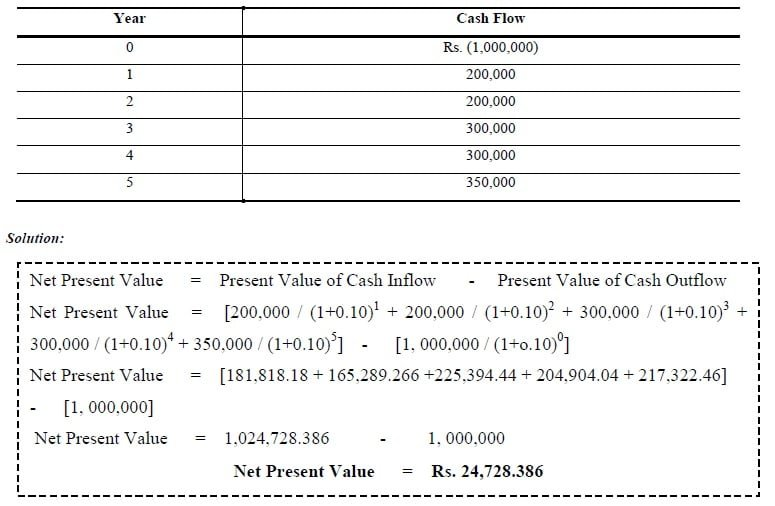Select Page

# Net Present Value (NPV)

The Net Present Value (NPV) of a project is the sum of all present values of cash inflows that are expected to occur over the life of project minus present values of all cash outflows. So we can say that Net Present Value is difference between Cash Inflow and Cash Outflow. If NPV > 0 the investment would add value to the firm the project may be accepted:

• NPV < 0 the investment would subtract value from the firm the project should be rejected
• NPV = 0 the investment would neither profit nor lose value for the firmExample 1: Find the Net Present Value assuming cost of capital is 10 %?(Acceptance Criterion: The higher the NPV the better the project)